# 9.A

9.A to attend more than 20 students but fewer than 40 students. A third of the pupils wrote a math test to mark 1, the sixth to mark 2, the ninth to mark 3. No one gets mark 4. How many students of class 9.A wrote a test to mark 5?

n =  14

### Step-by-step explanation: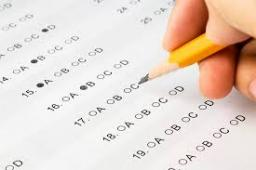Did you find an error or inaccuracy? Feel free to write us. Thank you!Tips to related online calculators
Do you want to calculate least common multiple two or more numbers?
Do you want to calculate greatest common divisor two or more numbers?

## Related math problems and questions:

• Class 9AOn the final certificate have one quarter of the class 9A mark "C" of mathematics, seventh mark "C" from the Czech language and two students failed in chemistry. How many students attend class 9A?
• LCD 2The least common denominator of 2/5, 1/2, and 3/4
• Shepherd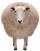The shepherd has fewer than 500 sheep; where they can be up to 2, 3, 4, 5, 6 row is always 1 remain, and as can be increased up to 7 rows of the sheep, and it is not increased no ovine. How many sheep has a shepherd?
• Four poplarsFour poplars are growing along the way. The distances between them are 35 m, 14 m, and 91 m. At least how many poplars need to be dropped to create the same spacing between the trees? How many meters will it be?
• SchoolLess than 500 pupils attend school. When it is sorted into pairs, one pupil remains. Similarly, when sorted into 3, 4, 5 and 6 members team one remains. Sorted to seven members teams, no left behind. How many pupils are attending this school?
• The bar chartThe bar chart shows the results of the math test. How many% of pupils achieved approximately better results than the average of the class? Diagram: 1-10 pupils, 2-4 pupils, 3-6 pupils, 4-2 pupils, 5-4 pupils. (lowest result are better)
• SchoolsThree schools are attended by 678 pupils. To the first attend 21 students more and to the third 108 fewer students than to second school. How many students attend the schools?
• MO C–I–1 2018An unknown number is divisible by just four numbers from the set {6, 15, 20, 21, 70}. Determine which ones.
• Four classses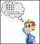Students of all 7, 8 and 9 classes in one school may take up 4,5,6 and 7 abreast and nobody will left. How many is the average count of pupils in one class if there are always four classes each grade?
• Buses 4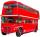intervals: 1st bus 40 min. 2nd bus 2h 3rd bud 20min How long take them to meet - as soon as possible?
• Lcm to eliminate yIdentify the least common multiple that would eliminate the y-variable. 6x - 5y = -4 4x + 2y = 28
• Hectares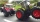The tractor plows the first day of 4.5 ha, the second day 6.3 ha, and the third day of 5.4 ha. It worked whole hours a day, and its hourly performance did not change and was the highest of the possible. How many hectares did it plow in one hour (what is i
• Marks at schoolThere are 30 students in the class. Five students in the class had a mark three triple at the end of the math certificate, and the other students had a mark of one or two. The average mark in all students' mathematics in the class at the end of the year w
• Coloured numbersMussel wrote four different natural numbers with coloured markers: red, blue, green and yellow. When the red number divides by blue, it gets the green number as an incomplete proportion, and yellow represents the remainder after this division. When it div
• School booksAt the beginning of the school year, the teacher distributed 480 workbooks and 220 textbooks. How many pupils could have the most in the classroom?
• Fewer than 500 sheep,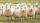There are fewer than 500 sheep, but if they stand in a double, triple, quadruple, five, and sixth-order, one sheep will remain. But they can stand in the seventh order. How many are sheep?
• Lcm 2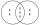Create the smallest possible number that is divisible by numbers 5,8,9,4,3